Courses

# CMOS Inverter Characteristics Electrical Engineering (EE) Notes | EduRev

## VLSI System Design

Created by: Machine Experts

## Electrical Engineering (EE) : CMOS Inverter Characteristics Electrical Engineering (EE) Notes | EduRev

The document CMOS Inverter Characteristics Electrical Engineering (EE) Notes | EduRev is a part of the Electrical Engineering (EE) Course VLSI System Design.
All you need of Electrical Engineering (EE) at this link: Electrical Engineering (EE)

Objectives

In this lecture you will learn the following

• CMOS Inverter Characterisitcs
• Noise Margins
• Regions of operation
• Beta-n by Beta-p ratio

15. CMOS Inverter Characterisitcs

The complementry CMOS inverter is realized by the series connection of a p- and n-device as in fig 15.11.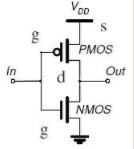Fig 15.11: CMOS Inverter

Inverter characteristics:
In the below graphical representation(fig.2.) The I-V characteristics of the p-device is reflected about x-axis. This step is followed by taking the absolute values of the p-device, Vds and superimposing the two characteristics. Solving Vinn and Vinp and Idsn = Idsp gives the desired transfer characteristics of a CMOS inverter as in fig3.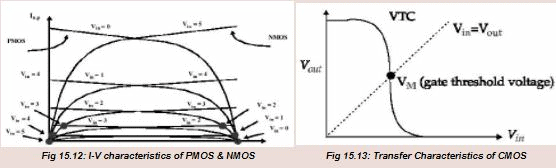15.2 Noise Margins

Noise margin is a parameter closely related to the input-output voltage characteristics. This parameter allows us to determine the allowable noise voltage on the input of a gate so that the output will not be affected. The specification most commonly
used to specify noise margin (or noise immunity) is in terms of two parameters- The LOW noise margin, NML, and the HIGH noised margin, NMH. With reference to Fig 4. NML is defined as the difference in magnitude between the maximum LOW output
voltage of the driving gate and the maximum input LOW voltage recognized by the driven gate. Thus,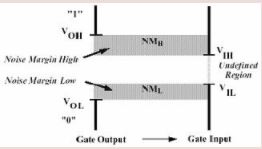Fig 15.2: Noise Margin diagram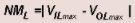The value of NMH is difference in magnitude between the minimum HIHG output voltage of the driving gate and the minimum input HIGH voltage recognized by the receiving gate. Thus,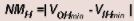Where,

VIHmin = minimum HIGH input voltage
VILmax = maximum LOW input voltage
VOHmin = minimum HIGH output voltage
VOLmax = maximum LOW output voltage.

15.3: Regions of Operation

The operation of CMOS inverter can be divided into five regions .The behavior of n- and p-devices in each of region may be found using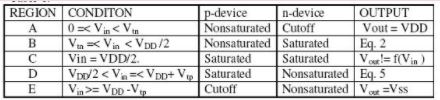We will describe about each regions in details-

Region A : This region is defined by 0 =< Vin < Vtn in which the n-device is cut off (Idsn =0), and the p-device is in the linear region. Since Idsn = –IIdsp, the drain-to-source current Idsp for the p-device is also zero. But for Vdsp = Vout– VDD, with Vdsp = 0, the output voltage is Vout=VDD.

Region B : This region is characterized by Vtn =< Vin < VDD /2 in which the p-device is in its nonsaturated region (Vds != 0) while the n-device is in saturation. The equivalent circuit for the inverter in this region can be represented by a resistor for the p-transistor and a current source for the n-transistor as shown in fig. 6 . The saturation current Idsn for the n-device is obtained by setting Vgs = Vin . This results in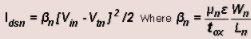and Vtn =threshold voltage of n-device, µn=mobility of electrons Wn = channel width of n-device & Ln = channel length of n-device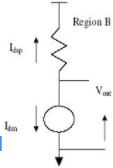Fig 15.31: Equivalent circuit of MOSFET in region B

The current for the p-device can be obtained by noting that Vgs =( Vin – VDD ) and Vds = (Vout – VDD ). And therefore,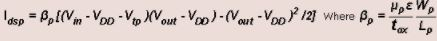and Vtp =threshold voltage of n-device, µp=mobility of electrons, Wp = channel width of n-device & Lp = channel length of n-device. The output voltage Vout can be expressed as-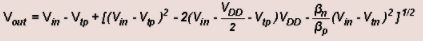Region C: In this region both the n- and p-devices are in saturation. This is represented by fig 7 which shows two current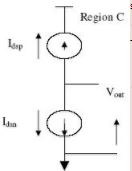Fig 15.32: Equivalent circuit of MOSFET in region C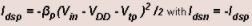This yields,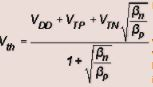By setting,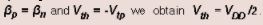Which implies that region C exists only for one value of Vin. We have assumed that a MOS device in saturation behaves like an ideal current soured with drain-to-source current being independent of Vds.In reality, as Vds increases, Ids also increases slightly; thus region C has a finite slope. The significant factor to be noted is that in region C, we have two current sources in series, which is an “unstable” condition.

Thus a small input voltage as a large effect at the output. This makes the output transition very steep, which contrasts with the equivalent nMOS inverter characteritics. characteritics. The above

expression of Vth is particularly useful since it provides the basis for defining the gate threshold Vinv which corresponds to the state where Vout=Vin .This region also defines the “gain” of the CMOS inverter when used as a small signal amplifier.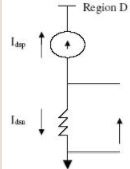Fig 15.33: Equivalent circuit of MOSFET in region D

Region D: This region is described by VDD/2 <Vin =< VDD+ Vtp.The p-device is in saturation while the n-device is operation in its nonsaturated region. This condition is represented by the equivalent circuit shown in fig 15.33 .The two currents may be written as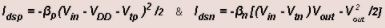with Idsn = -Idsp.

The output voltage becomes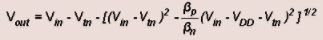Region E: This region is defined by the input condition Vin >= VDD -Vtp, in which the pdevice is cut off (Idsp =0), and the n-device is in the linear mode. Here, Vgsp= Vin - VDD Which is more positive than Vtp. The output in this region is Vout=0

From the transfer curve , it may be seen that the transition between the two states is very step.This characteristic is very desirable because the noise immunity is maximized.

15.4 βn/βp ratio: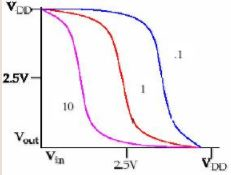Figure 15.4: βnp graph

The gate-threshold voltage, Vinv, where Vin =Vout is dependent onβn/βp . Thus, for given process, if we want to change βn/βp we need to change the channel dimensions, i.e.,channel-length L and channel-width W. Therefore it can be seen that as the ratio βn/βp is decreased, the transition region shifts from left to right; however, the output voltage transition remains sharp.

Offer running on EduRev: Apply code STAYHOME200 to get INR 200 off on our premium plan EduRev Infinity!

35 docs|28 tests

,

,

,

,

,

,

,

,

,

,

,

,

,

,

,

,

,

,

,

,

,

;